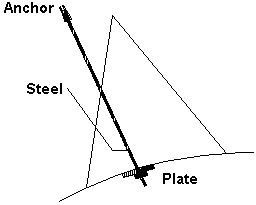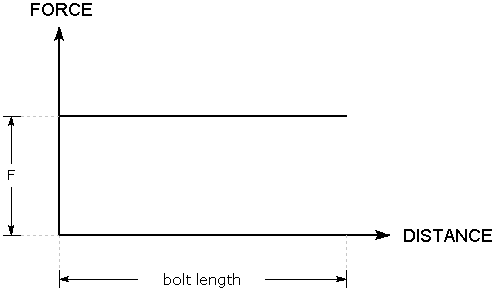The Rocscience International Conference 2021 Proceedings are now available. Read Now

# Mechanically Anchored

The effective tensile capacity of a Mechanically Anchored bolt in RocPlane is dictated by the weakest of the following components, as shown in the figure below:

• Anchor Capacity
• Steel tensile capacityTherefore, when you enter these parameters in the Bolt Properties dialog, only the lowest-valued parameter is used in the analysis. The other two values are not used.

## Bolt Force Diagram

When a Mechanically Anchored bolt intersects a wedge plane, it does not matter where the wedge plane intersects the bolt. The same force is applied to the wedge regardless of the point of intersection along the length of the bolt.

F = min ( f1, f2, f3 )

where f1, f2, and f3 are the anchor, steel, and plate capacities, respectively.

The Bolt Force Diagram for a Mechanically Anchored bolt is simply a horizontal line representing a constant value of applied force, regardless of where a wedge plane intersects the bolt.